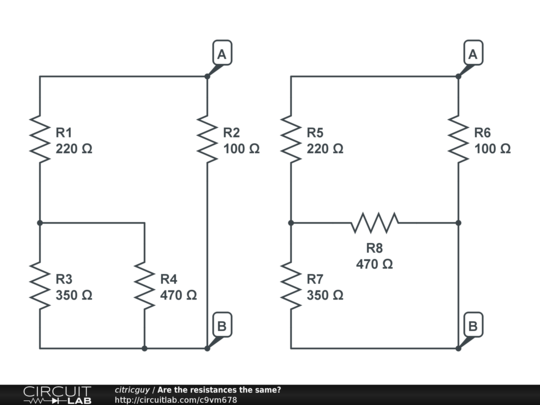Why doesn't the relocation of this resistor affect the circuit's total resistance?

Why does moving the 470Ω resistor in these examples have no effect on the total resistance between A and B?This is a new question regarding the answer given here by @helloworld992.

• – user17592 Mar 25 '13 at 7:40
• The circuits are functionally identical. Even the node label B points to exactly the same thing in both schematics: the junction of R2, R3 and R4. If you read a lot of schematics, your brain will slowly become "blind" to seeing these kinds of things as different. – Kaz Mar 25 '13 at 8:24
• I don't understand why you essentially ask the same question after your original one has been answered properly. This is nearly a duplicate. – Rev1.0 Mar 25 '13 at 15:37
• There is no 'resistor relocation' here, electrically speaking. – user207421 Apr 10 '13 at 23:12

470Ω is connected to node B in either picture.

Wires are assumed ideal conductors. Both circuits are identical. They have the same topology. They are only drawn differently.

Simply because you're not actually moving the resistor in the circuit.

The wire running past point "B" is a 0Ω connection. As such, no matter what side of B you connect R8 to, it's still effectively the same circuit.

I've redrawn the two circuits with the interesting connections pulled away from their node. Since anything joined by a wire is considered to be at the same potential, this should illustrate that it doesn't matter where the other end of R16 is connected.I've added rather more wire than is necessary, but it should help reinforce the idea that you can connect anywhere on the red traces.

your drawing the same circuit but with different drawings, B on both drawing is connected to directly (or through zero resistance wire) to the same node

Two schematics of one circuit. Both are the same ...